# NCERT Solutions for Class 9 Maths Chapter 12 Heron's Formula| PDF Download

On this page you will get Chapter 12 Heron's Formula NCERT Solutions for Class 9 Maths that will useful in the preparation of examinations and make you aware of concepts of the chapter. If you want to study offline then you can also Download PDF of NCERT Solutions for Class 9 Maths Chapter 12 Heron's Formula. You can also complete your homework on time through the help of these Chapter 12 NCERT Solutions and able to solve the difficult problems given in a exercise.

NCERT Solutions for Class 9 Maths is good way through which one can build their basic knowledge and getting good marks in the examination. These NCERT Solutions are updated according to the latest NCERT Class 9 Maths textbook and syllabus.Page No: 202

Exercise 12.1

1. A traffic signal board, indicating 'SCHOOL AHEAD', is an equilateral triangle with side 'a'. Find the area of the signal board, using Heron’s formula. If its perimeter is 180 cm, what will be the area of the signal board?

Length of the side of equilateral triangle = a
Perimeter of the signal board = 3a = 180 cm
∴ 3a = 180 cm ⇒ a = 60 cm
Semi perimeter of the signal board (s) = 3a/2
Using heron's formula,
Area of the signal board = √s (s-a) (s-b) (s-c)
= √(3a/2) (3a/2 - a) (3a/2 - a) (3a/2 - a)
= √3a/2 × a/2 × a/2 × a/2
= √3a4/16
= √3a2/4
= √3/4 × 60 × 60 = 900√3 cm2

2. The triangular side walls of a flyover have been used for advertisements. The sides of the walls are 122 m, 22 m and 120 m (see Fig. 12.9). The advertisements yield an earning of ₹5000 per m2 per year. A company hired one of its walls for 3 months. How much rent did it pay?The sides of the triangle are 122 m, 22 m and 120 m.
Perimeter of the triangle is 122 + 22 + 120 = 264m
Semi perimeter of triangle (s) = 264/2 = 132 m
Using heron's formula,
= √132(132 - 122) (132 - 22) (132 - 120) m2
= √132 × 10 × 110 × 12 m2
= 1320 m2
Rent of advertising per year = ₹ 5000 per m2
Rent of one wall for 3 months = ₹ (1320 × 5000 × 3)/12 = ₹ 1650000

Page No: 203

3. There is a slide in a park. One of its side walls has been painted in some colour with a message “KEEP THE PARK GREEN AND CLEAN” (see Fig. 12.10 ). If the sides of the wall are 15 m, 11 m and 6 m, find the area painted in colour.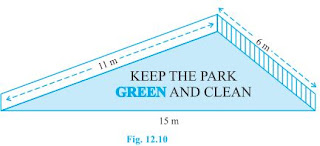Sides of the triangular wall are 15 m, 11 m and 6 m.
Semi perimeter of triangular wall (s) = (15 + 11 + 6)/2 m = 16 m
Using heron's formula,
Area of the message = √s (s-a) (s-b) (s-c)
= √16(16 - 15) (16 - 11) (16 - 6) m2
= √16 × 1 × 5 × 10 m2 = √800 m2
= 20√2 m2

4. Find the area of a triangle two sides of which are 18cm and 10cm and the perimeter is 42cm.

Two sides of the triangle = 18cm and 10cm
Perimeter of the triangle = 42cm
Third side of triangle = 42 - (18+10) cm = 14cm
Semi perimeter of triangle = 42/2 = 21cm
Using heron's formula,
Area of the triangle = √s (s-a) (s-b) (s-c)
= √21(21 - 18) (21 - 10) (21 - 14) cm2
= √21 × 3 × 11 × 7 m2
= 21√11 cm2

5. Sides of a triangle are in the ratio of 12 : 17 : 25 and its perimeter is 540cm. Find its area.

Ratio of the sides of the triangle = 12 : 17 : 25
Let the common ratio be x then sides are 12x, 17x and 25x
Perimeter of the triangle = 540cm
12x + 17x + 25x = 540 cm
⇒ 54x = 540cm
⇒ x = 10
Sides of triangle are,
12x = 12 × 10 = 120cm
17x = 17 × 10 = 170cm
25x = 25 × 10 = 250cm
Semi perimeter of triangle(s) = 540/2 = 270cm
Using heron's formula,
Area of the triangle = √s (s-a) (s-b) (s-c)
= √270(270 - 120) (270 - 170) (270 - 250)cm2
= √270 × 150 × 100 × 20 cm2
= 9000 cm2

6. An isosceles triangle has perimeter 30 cm and each of the equal sides is 12 cm. Find the area of the triangle.

Length of the equal sides = 12cm
Perimeter of the triangle = 30cm
Length of the third side = 30 - (12+12) cm = 6cm
Semi perimeter of the triangle(s) = 30/2 cm = 15cm
Using heron's formula,
Area of the triangle = √s (s-a) (s-b) (s-c)
= √15(15 - 12) (15 - 12) (15 - 6)cm2
= √15 × 3 × 3 × 9 cm2
= 9√15 cm2

Page No: 206

Exercise 12.2

1. A park, in the shape of a quadrilateral ABCD, has ∠C = 90º, AB = 9 m, BC = 12 m, CD = 5 m and AD = 8 m. How much area does it occupy?

∠C = 90º, AB = 9 m, BC = 12 m, CD = 5 m and AD = 8 m
BD is joined.In ΔBCD,
By applying Pythagoras theorem,
BD2 = BC2 + CD2
⇒ BD2 = 122 + 52
⇒ BD2 = 169
⇒ BD = 13 m
Area of ΔBCD = 1/2 × 12 × 5 = 30 m2
Now,
Semi perimeter of ΔABD(s) = (8 + 9 + 13)/2 m = 30/2 m = 15 m
Using heron's formula,
Area of ΔABD  = √s (s-a) (s-b) (s-c)
= √15(15 - 13) (15 - 9) (15 - 8) m2
= √15 × 2 × 6 × 7 m2
= 6√35 m2 = 35.5 m2 (approx)

Area of quadrilateral ABCD = Area of ΔBCD + Area of ΔABD = 30 m+ 35.5m2 = 65.5m

2. Find the area of a quadrilateral ABCD in which AB = 3 cm, BC = 4 cm, CD = 4 cm, DA = 5 cm and AC = 5 cm.

AB = 3 cm, BC = 4 cm, CD = 4 cm, DA = 5 cm and AC = 5 cm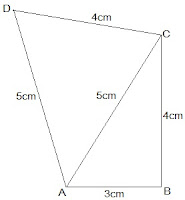In ΔABC,
By applying Pythagoras theorem,
AC2 = AB2 + BC2
⇒ 52 = 32 + 42
⇒ 25 = 25
Thus, ΔABC is a right angled at B.
Area of ΔBCD = 1/2 × 3 × 4 = 6 cm2
Now,
Semi perimeter of ΔACD(s) = (5 + 5 + 4)/2 cm = 14/2 cm = 7 m
Using heron's formula,
Area of ΔABD  = √s (s-a) (s-b) (s-c)
= √7(7 - 5) (7 - 5) (7 - 4) cm2
= √7 × 2 × 2 × 3 cm2
= 2√21 cm2 = 9.17 cm2 (approx)

Area of quadrilateral ABCD = Area of ΔABC + Area of ΔABD = 6 cm+ 9.17 cm2 = 15.17 cm

3. Radha made a picture of an aeroplane with coloured paper as shown in Fig 12.15. Find the total area of the paper used.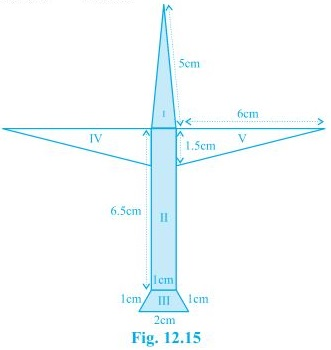Length of the sides of the triangle section I = 5cm, 1cm and 5cm
Perimeter of the triangle = 5 + 5 + 1 = 11cm
Semi perimeter = 11/2 cm = 5.5cm
Using heron's formula,
Area of section I  = √s (s-a) (s-b) (s-c)
= √5.5(5.5 - 5) (5.5 - 5) (5.5 - 1) cm2
= √5.5 × 0.5 × 0.5 × 4.5 cm2
= 0.75√11 cm2 = 0.75 × 3.317cm2 = 2.488cm2 (approx)
Length of the sides of the rectangle of section I = 6.5cm and 1cm
Area of section II = 6.5 × 1 cm2 =  6.5 cm2
Section III is an isosceles trapezium which is divided into 3 equilateral of side 1cm each.
Area of the trapezium = 3 × √3/4 × 1cm= 1.3 cm2 (approx)
Section IV and V are 2 congruent right angled triangles with base 6cm and height 1.5cm
Area of region IV and V = 2 × 1/2 × 6 × 1.5cm= 9cm2
Total area of the paper used = (2.488 + 6.5 + 1.3 + 9)cm= 19.3 cm2

4. A triangle and a parallelogram have the same base and the same area. If the sides of the triangle are 26 cm, 28 cm and 30 cm, and the parallelogram stands on the base 28 cm, find the height of the parallelogram.

Given,
Area of the parallelogram and triangle are equal.Length of the sides of the triangle are 26 cm, 28 cm and 30 cm.
Perimeter of the triangle = 26 + 28 + 30 = 84 cm
Semi perimeter of the triangle = 84/2 cm = 42 cm
Using heron's formula,
Area of the triangle = √s (s-a) (s-b) (s-c)
= √42(42 - 26) (46 - 28) (46 - 30) cm2
= √46 × 16 × 14 × 16 cm2
= 336 cm2Let height of parallelogram be h.
Area of parallelogram = Area of triangle
28cm × h = 336 cm2
h = 336/28 cm
h = 12 cm
The height of the parallelogram is 12 cm.

Page No: 207

5. A rhombus shaped field has green grass for 18 cows to graze. If each side of the rhombus is 30 m and its longer diagonal is 48 m, how much area of grass field will each cow be getting?

Diagonal AC divides the rhombus ABCD into two congruent triangles of equal area.Semi perimeter of ΔABC = (30 + 30 + 48)/2 m = 54 m
Using heron's formula,
Area of the ΔABC = √s (s-a) (s-b) (s-c)
= √54(54 - 30) (54 - 30) (54 - 48) m2
= √54 × 24 × 24 × 6 cm2
= 432 m2
Area of field = 2 × area of the ΔABC = (2 × 432)m= 864 m2
Thus,
Area of grass field which each cow will be getting = 864/18 m= 48 m2

6. An umbrella is made by stitching 10 triangular pieces of cloth of two different colours (see Fig.12.16), each piece measuring 20 cm, 50 cm and 50 cm. How much cloth of each colour is required for the umbrella?Semi perimeter of each triangular piece = (50 + 50 + 20)/2 cm = 120/2 cm = 60cm
Using heron's formula,
Area of the triangular piece = √s (s-a) (s-b) (s-c)
= √60(60 - 50) (60 - 50) (60 - 20) cm2
= √60 × 10 × 10 × 40 cm2
= 200√6 cm2
Area of triangular piece = 5 × 200√6 cm2 = 1000√6 cm2

7. A kite in the shape of a square with a diagonal 32 cm and an isosceles triangle of base 8 cm and sides 6 cm each is to be made of three different shades as shown in Fig. 12.17. How much paper of each shade has been used in it?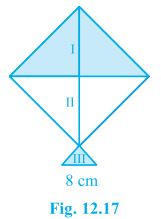We know that,
As the diagonals of a square bisect each other at right angle.
Area of given kite = 1/2 (diagonal)2
= 1/2 × 32 × 32 = 512 cm2
⇒ 512/2 cm= 256 cm2
So, area of paper required in each shade = 256 cm2
For the III section,
Length of the sides of triangle = 6cm, 6cm and 8cm
Semi perimeter of triangle = (6 + 6 + 8)/2 cm = 10cm
Using heron's formula,
Area of the III triangular piece = √s (s-a) (s-b) (s-c)
= √10(10 - 6) (10 - 6) (10 - 8) cm2
= √10 × 4 × 4 × 2 cm2
= 8√6 cm2

8. A floral design on a floor is made up of 16 tiles which are triangular, the sides of the triangle being 9 cm, 28 cm and 35 cm (see Fig. 12.18). Find the cost of polishing the tiles at the rate of 50p per cm2 .Semi perimeter of the each triangular shape = (28 + 9 + 35)/2 cm = 36 cm
Using heron's formula,
Area of the each triangular shape = √s (s-a) (s-b) (s-c)
= √36(36 - 28) (36 - 9) (36 - 35) cm2
= √36 × 8 × 27 × 1 cm2
= 36√6 cm= 88.2 cm2
Total area of 16 tiles = 16 × 88.2 cm2 = 1411.2 cm2Cost of polishing tiles = 50p per cm2
Total cost of polishing the tiles = Rs. (1411.2 × 0.5) = Rs. 705.6

9. A field is in the shape of a trapezium whose parallel sides are 25 m and 10 m. The non-parallel sides are 14 m and 13 m. Find the area of the field.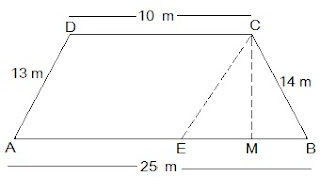Let ABCD be the given trapezium with parallel sides AB = 25m and CD = 10mand the non-parallel sides AD = 13m and BC = 14m.
CM ⊥ AB and CE || AD.
In ΔBCE,
BC = 14m, CE = AD = 13 m and
BE = AB - AE = 25 - 10 = 15m
Semi perimeter of the ΔBCE = (15 + 13 + 14)/2 m = 21 m
Using heron's formula,
Area of the ΔBCE = √s (s-a) (s-b) (s-c)
= √21(21 - 14) (21 - 13) (21 - 15) m2
= √21 × 7 × 8 × 6 m2
= 84 m2
also, area of the ΔBCE = 1/2 × BE × CM = 84 m2
⇒ 1/2 × 15 × CM = 84 m2
⇒ CM = 168/15 m
⇒ CM = 56/5 m
Area of the parallelogram AECD = Base × Altitude = AE × CM = 10 × 84/5 = 112 m2
Area of the trapezium ABCD = Area of AECD + Area of ΔBCE
= (112+ 84) m= 196 m

## NCERT Solutions for Class 9 Maths Chapter 12 Heron's Formula

Chapter 12 Heron's Formula NCERT Solutions is a great way through which you can find area of triangles. You know area of a triangles is 1/2 × base × height. When it is not possible to find the height of the triangle easily and measures of all the three sides are known then we use Heron’s formula.

• Area of a Triangle — by Heron’s Formula: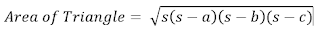where a, b and c are the sides of the triangle, and s = semi-perimeter, i.e., half the perimeter of the triangle = a+b+c/2

• Application of Heron’s Formula in Finding Areas of Quadrilaterals: We will extend the use of Heron's Formula to find the area of quadrilaterals. We can divide the quadrilateral in triangular parts and then use the formula for area of the triangle.

There are only two exercises in chapter 12 Heron's Formula where you will learn to grasp key concepts of the chapter properly. Below you can find exercisewise NCERT Solutions of Heron's Formula just by clicking on the links.

Studyrankers experts have prepared these Chapter 12 NCERT Solutions that are detailed and accurate through which a student can clear their doubts easily. By solving the questions from Class 9 NCERT textbook, a student will gain confidence that is going to help them in exams.

### NCERT Solutions for Class 9 Maths Chapters:

 Chapter 1 Number Systems Chapter 2 Polynomials Chapter 3 Coordinate Geometry Chapter 4 Linear Equations in Two Variables Chapter 5 Introduction to Euclid’s Geometry Chapter 6 Lines and Angles Chapter 7 Triangles Chapter 8 Quadrilaterals Chapter 9 Areas of Parallelograms and Triangles Chapter 10 Circles Chapter 11 Constructions Chapter 13 Surface Areas And Volumes Chapter 14 Statistics Chapter 15 Probability

FAQ on Chapter 12 Heron's Formula

#### What are the benefits of NCERT Solutions for Chapter 12 Heron's Formula Class 9 NCERT Solutions?

NCERT Solutions are very important in improving problem skills and understand all the important points of the chapter. Here we have detailed every solutions step by step so you can easily know the concepts.

#### How many exercises in Chapter 12 Heron's Formula?

Only two exercises are there in the chapter 12 Heron's Formula. In the first exercise, you need to find the area of triangles by using heron's formula. In the second exercise, you have to find the area of quadrilaterals. For finding area of a quadrilateral we divide it into various triangles. After we need to use Heron’s formula to find the area of the triangles.

#### Find the length of a diagonal of a square whose side is 2 cm.

The diagonal of a square = (√2) a cm
∴ Length of the diagonal = (√2) x 2 cm = 2√2cm.

#### Find the height of an equilateral triangle whose side is 2 cm.

Since height of an equilateral triangle is given by
Height = (√3/2) x side
⇒ height =(√3/2) x 2 cm = 3 cm.